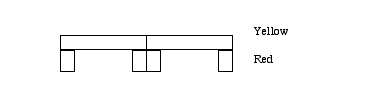# Assessment Teacher Directed

## Bridge Construction

### Topic - Algebra - Patterns and Functions

Teacher Directions:

1. Students work alone using Cuisenaire rods to construct bridges and discover a patterns.
2. Give each person an assortment of rods and the following work sheet.
3. Explain that they are to build different kinds of bridges and answer some questions about them.
4. Tell them when they are finished to ... and when all are done or after 40 minutes they will discuss the results as a class.

Student work sheet:

## Bridge Construction

### Topic - Patterns and Functions

Directions:
Part 1

You are an engineer who wants to build different kinds of bridges. The first bridge is a one span bridge. An example can be made with one yellow rod and two red.

Build a bridge with a yellow rod as a span and red rods as piers.

The bridge is 5 cm

To make the bridge longer a second span can be added with another yellow rod and two red rods.

This makes the bridge with a 10 cm span:As you build the bridge longer think of a way to keep track of the number of spans (yellow rods) and piers (red rods) needed to make bridges of different lengths.

Your goal is to find a pattern for the number of spans and piers (rods of each color) needed to build a bridge of any size. You can use the following sequence as a guide.

Build a three-span bridge.

1. How many spans (yellow rods) did you use? _____
2. How long is your bridge? _____
3. How many piers (red rods) did you use? _____
4. How many spans and piers (rods) did you use altogether? _____

Try to answer these questions without building a five-span bridge. Later, if you want, you can build a five-span bridge to check your answers.

1. How many spans (yellow rods) would you need for a five-span bridge? _____
2. How long would your bridge be? _____
3. cHow many piers (red rods) would you need? _____
4. How many spans and piers (rods) would you need altogether? _____

3. Without building a twelve-span bridge, answer the following questions.

1. How many spans (yellow rods) would you need for a twelve-span bridge? _____
2. How long would your bridge be? _____
3. How many piers (red rods) would you need? _____
4. How many spans and piers (rods) would you need altogether? _____

4. How many spans (yellow rods) and piers (red rods) would you need to build a twenty-eight-span bridge?

_____ spans (yellow rods) and _____ piers (red rods).

5. Write a rule for figuring the total number of spans and piers (rods) you would need to build a bridge if you knew how many spans the bridge had.

6. How many spans (yellow rods) and piers (red rods) would you need to build a bridge that is 185 cm long? _____ spans (yellow rods) and _____ piers (red rods).

Part 2

The bridges for this part are built like this 2 - span bridge:The black rods are 7 cm long, and the light green rods are 3 cm long. Notice that the supports are shared between spans, except at the ends.

1. Build a three-span bridge of this same kind, with black and light green.

a. How many spans (black rods) did you use? _____

b. How long is your bridge? _____

c. How many piers (light green rods) did you use? _____

d. How many spans and piers (rods) did you use all together? _____

2. Try to answer these questions without building a five-span bridge. If you want, build a five-span bridge to check your answers.

a. How many spans (black rods) would you need for a five-span bridge? _____

b. How long would your bridge be? _____

c. How many piers (light green rods) would you need? _____

d. How many spans and piers (rods) would you need altogether? _____

3. Without building a thirteen-span bridge, answer the following questions.

a. How many spans (black rods) would you need for a thirteen-span bridge? _____

b. How long would your bridge be? _____

c. How many piers (light green rods) would you need? _____

d. How many spans and piers (rods) would you need altogether? _____

4. How many spans (black rods) and piers (light green rods) would you need to build a fifty-six-span bridge? _____ spans (black rods) and _____ piers (light green rods). Explain your answer.

5. Write a rule for figuring how many spans and piers (rods) you would need to build a bridge if you know how many spans the bridge had.

6. How many spans (black rods) and piers (light green rods) would you need to build a bridge that is at least 429 cm long? _____ spans (black rods) and _____ piers (light green rods). Explaining your answer.